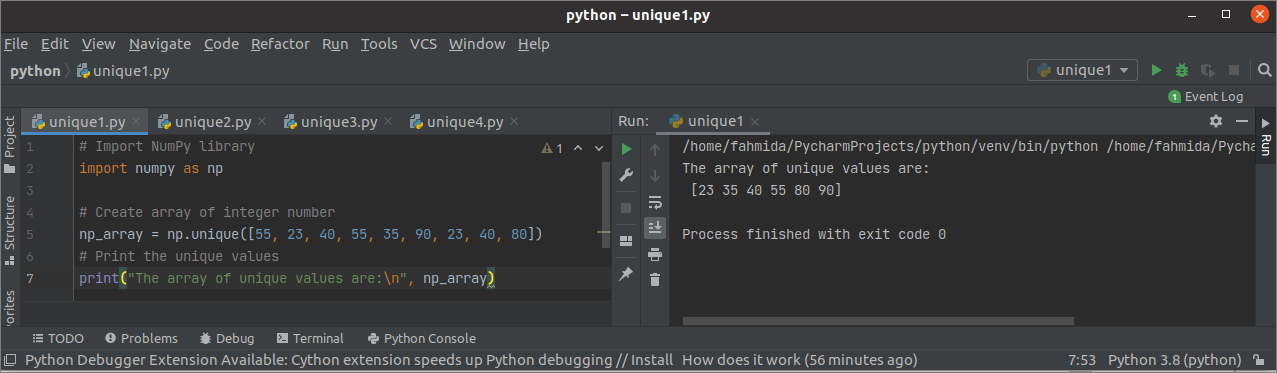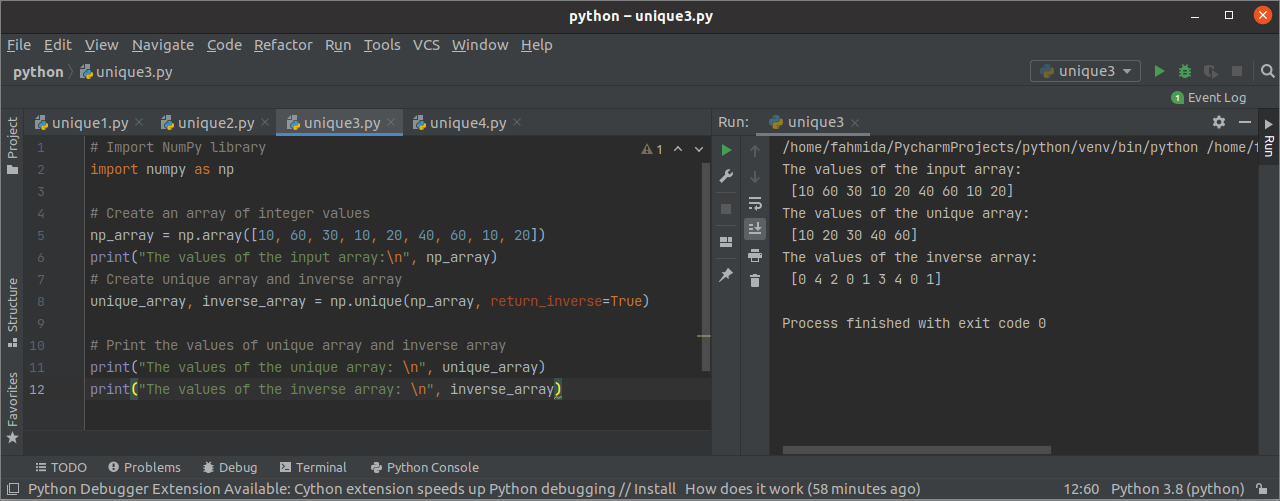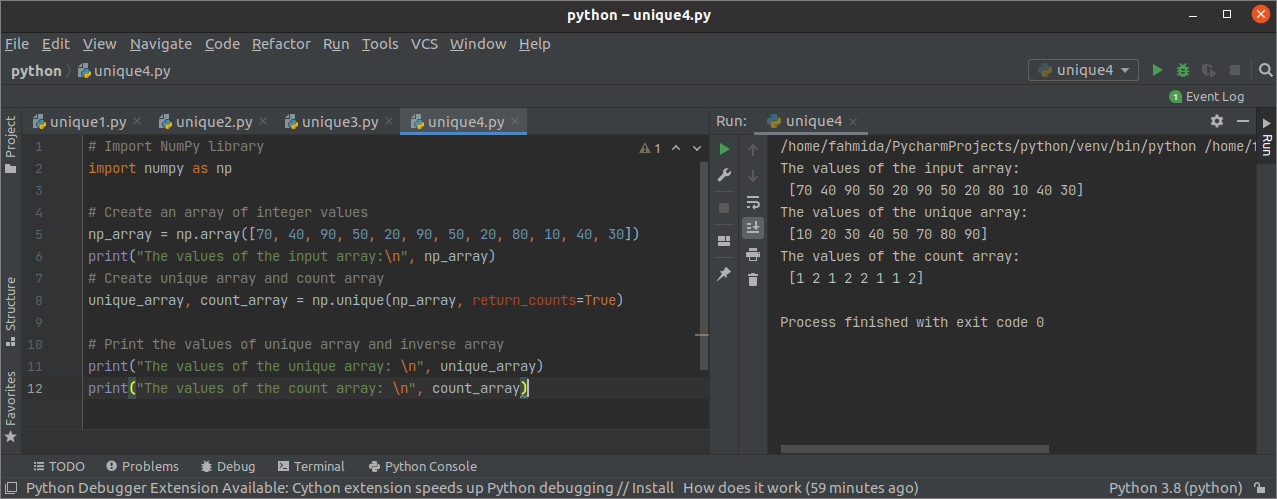# How to Use Python NumPy unique() Function

NumPy library is used in python to create one or more dimensional arrays, and it has many functions to work with the array. The unique() function is one of this library’s useful functions to find out the unique values of an array and return the sorted unique values. This function can also return a tuple of array values, the array of the associative indices, and the number of times each unique value appears in the main array. The different uses of this function are shown in this tutorial.

## Syntax:

The syntax of this function is given below.

array numpy.unique(input_array, return_index, return_inverse, return_counts, axis)

This function can take five arguments, and the purpose of these arguments is explained below.

• input_array: It is a mandatory argument that contains the input array from which the output array will be returned by retrieving the unique values. If the array is not a one-dimensional array, then the array will be flattened.

• return_index: It is an optional argument that can take a Boolean value. If this argument’s value is set to True, it will return the input array’s indices.

• return_inverse: It is an optional argument that can take a Boolean value. If this argument’s value is set to True, then it will return the indices of the output array that contains the unique values.

• return_counts: It is an optional argument that can take a Boolean value. If this argument’s value is set to True, then it will return the number of times each element of the unique array appears in the input array.

• axis: It is an optional argument that can take any integer value or None. If no value is set for this argument, then the input array will be flattened.

The unique() function can return four types of arrays based on the argument values.

## Example-1: Print the unique values of the one-dimensional array

The following example shows the use of the unique() function to create an array with the unique values of a one-dimensional array. A one-dimensional array of 9 elements has been used as the unique() function’s argument value. The returned value of this function has printed later.

# Import NumPy library

import numpy as np

# Create array of an integer number

np_array = np.unique([55, 23, 40, 55, 35, 90, 23, 40, 80])

# Print the unique values

print("The array of unique values are:
"
, np_array)

Output:

The following output will appear after executing the above script. The input array contains 6 unique elements that are shown in the output.## Example-2: Print the unique values and indices based on the input array

The following example shows how the unique values and indices of the two-dimensional array can be retrieved using the unique() function. A two-dimensional array of 2 rows and 6 columns has been used as the input array. The value of the return_index argument has been set to True to get the input array indices based on the unique array values.

# Import NumPy library

import numpy as np

# Create a two-dimensional array

np_array = np.array([[6, 4, 9, 6, 2, 9], [3, 7, 7, 6, 1, 3]])

# Print the two-dimensional array

print("The content of the two-dimensional array:
"
, np_array)

# Create the unique array and the index array of unique values

unique_array, index_array = np.unique(np_array, return_index=True)

# Print the values of unique and index arrays

print("The content of the unique array:
"
, unique_array)

print("The content of the index array:
"
, index_array)

Output:

The following output will appear after executing the above script. The input array contains 7 unique values. The output shows the array of 7 unique values and 7 indices of those values from the input array.

## Example-3: Print the unique values and indices based on the output array

The following example shows how the unique values of a one-dimensional array and the indices based on the unique values by using the unique() function. In the script, a one-dimensional array of 9 elements has used as the input array. The value of the return_inverse argument is set to True that will return another array of indices based on the unique array index. Both unique array and index array have printed later.

# Import NumPy library

import numpy as np

# Create an array of integer values

np_array = np.array([10, 60, 30, 10, 20, 40, 60, 10, 20])

print("The values of the input array:
"
, np_array)

# Create the unique array and inverse array

unique_array, inverse_array = np.unique(np_array, return_inverse=True)

# Print the values of the unique array and inverse array

print("The values of the unique array:
"
, unique_array)

print("The values of the inverse array:
"
, inverse_array)

Output:

The following output will appear after executing the above script. The output showed the input array, unique array, and inverse array. The input array contains 5 unique values. These are 10, 20, 30, 40, and 60. The input array contains 10 in three indices that are the first element of the unique array. So, 0 has appeared three times in the inverse array. The other values of the inverse array have been placed in the same way.## Example-4: Print the unique values and the frequency of each unique value

The following example shows how the unique() function can retrieve the unique values and the frequency of each unique value of the input array. The value of the return_counts argument has been set to True for getting the array of frequency values. A one-dimensional array of 12 elements has been used in the unique() function as an input array. The array of unique values and the frequency values have been printed later.

# Import NumPy library

import numpy as np

# Create an array of integer values

np_array = np.array([70, 40, 90, 50, 20, 90, 50, 20, 80, 10, 40, 30])

print("The values of the input array:
"
, np_array)

# Create unique array and count array

unique_array, count_array = np.unique(np_array, return_counts=True)

# Print the values of the unique array and inverse array

print("The values of the unique array:
"
, unique_array)

print("The values of the count array:
"
, count_array)

Output:

The following output will appear after executing the above script. The input array, unique array, and count array have been printed in the output.## Conclusion

The detailed uses of unique() functions have been explained in this tutorial by using multiple examples. This function can return the values of different arrays and have shown here by using one-dimensional and two-dimensional arrays.

sources news post: linuxhint.com